In :
%pylab inline

Populating the interactive namespace from numpy and matplotlib

In :
from __future__ import division
from deltasigma import *


# NTF synthesis - demo #2¶

Demonstration of the simulateDSM function, as in the MATLAB Delta Sigma Toolbox, employing its Python port deltasigma.

• In the first section, the Noise Transfer Function (NTF) is synthesized for a 5th-order, low-pass modulator and for a 8th-order band-pass modulator.

• In each case, the modulator is simulated - with simulateDSM - and its output plotted in terms of time response and spectrum.
• The Signal to Noise Ratio (SNR) is evaluated from the spectrum through calculateSNR.
• The SNR for different amplitudes is predicted through predictSNR, simulated with simulateSNR and the peak values are extracted with peakSNR.
• In the second section we move on to the synthesis and simulation of a 7th-order low-pass multi-bit modulator.

## 5th-order low-pass modulator¶

General parameters and synthesis:

In :
OSR = 32
order = 5
H = synthesizeNTF(order, OSR, 1)


### Time-domain simulation with simulateDSM¶

A test sine wave is employed for the time simulation, having amplitude $A = .5$ (equiv. -6 dBFS) and a frequency equal to $f_{test} = 2/3 f_B$.

In :
figure(figsize=(20, 4))
N = 8192
fB = int(np.ceil(N/(2.*OSR)))
ftest = np.floor(2./3.*fB)
u = 0.5*np.sin(2*np.pi*ftest/N*np.arange(N))
v, xn, xmax, y = simulateDSM(u, H)
t = np.arange(301)
step(t, u[t],'r')
hold(True)
step(t, v[t], 'g')
axis([0, 300, -1.2, 1.2])
xlabel('Sample Number')
ylabel('u, v')
title('Modulator Input & Output');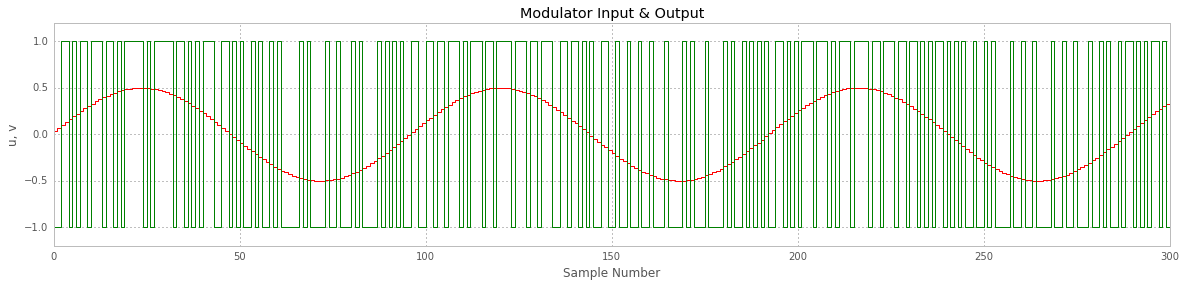### Output spectrum and extraction of the SNR with calculateSNR¶

From the above time simulation, the spectrum is computed - through direct FFT of the Hann-windowed time waveform.

The obtained spectrum (blue) is compared with the expected performances that can be computed evaluating the modulator transfer function in the frequency domain (magenta).

In :
f = np.linspace(0, 0.5, N/2. + 1)
spec = np.fft.fft(v * ds_hann(N))/(N/4)
plot(f, dbv(spec[:N/2. + 1]),'b', label='Simulation')
figureMagic([0, 0.5], 0.05, None, [-120, 0], 20, None, (16, 6), 'Output Spectrum')
xlabel('Normalized Frequency')
ylabel('dBFS')
snr = calculateSNR(spec[2:fB+1], ftest - 2)
text(0.05, -10, 'SNR = %4.1fdB @ OSR = %d' % (snr, OSR), verticalalignment='center')
NBW = 1.5/N
Sqq = 4*evalTF(H, np.exp(2j*np.pi*f)) ** 2/3.
hold(True)
plot(f, dbp(Sqq * NBW), 'm', linewidth=2, label='Expected PSD')
text(0.49, -90, 'NBW = %4.1E x $f_s$' % NBW, horizontalalignment='right')
legend(loc=4);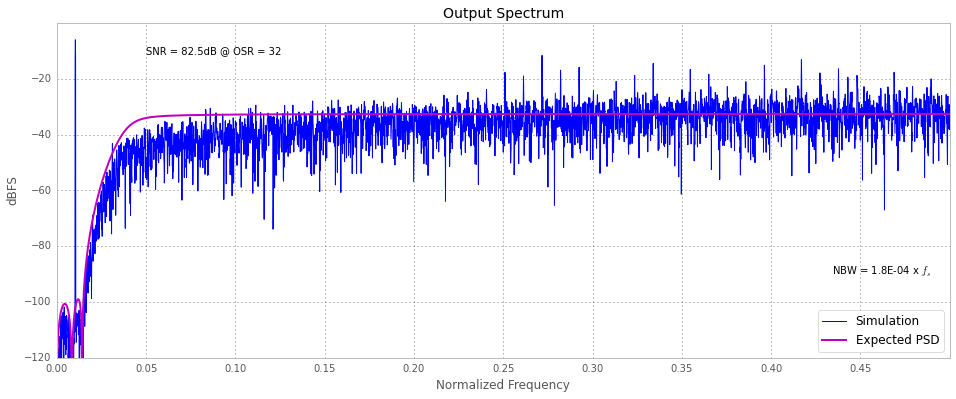### SNR vs input amplitude: prediction, simulation and peak extraction¶

Being the example modulator a binary (single-bit) structure, we can predict the SNR curve using the describing function method of Ardalan and Paulos. This is done with the function predictSNR.

Next, we check the agreement between the approximate - but very quick - method above and actual results obtained with extended time simulations of the modulator. This operation is performed by simulateSNR.

Lastly, we interpolate the simulation results close to the SNR peak, with peakSNR, to get an approximate value for peak SNR that can be ideally expected by the syntesized modulator structure and its corresponding amplitude value.

In :
snr_pred, amp_pred, _, _, _ = predictSNR(H, OSR)
snr, amp  = simulateSNR(H, OSR)

//anaconda/lib/python2.7/site-packages/scipy/signal/filter_design.py:1055: BadCoefficients: Badly conditioned filter coefficients (numerator): the results may be meaningless

In :
plot(amp_pred, snr_pred, '-', amp, snr, 'og-.')
figureMagic([-100, 0], 10, None, [0, 100], 10, None, (16, 6),'SQNR')
xlabel('Input Level (dBFS)')
ylabel('SQNR (dB)')
pk_snr, pk_amp = peakSNR(snr, amp)
text(-25, 85, 'peak SNR = %4.1fdB\n@ OSR = %d\n' % (pk_snr, OSR), horizontalalignment='right');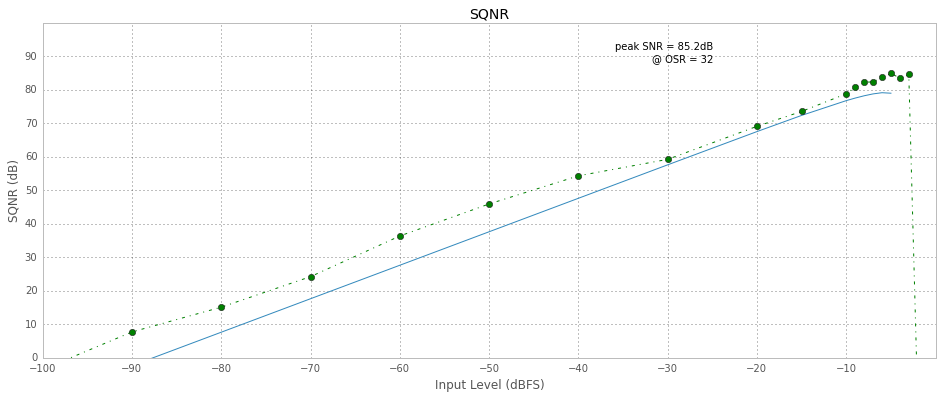### Bandpass Modulator¶

In :
f0 = 1./8
OSR = 64
order = 8
H = synthesizeNTF(order, OSR, 1, 1.5, f0)


### Time-domain simulation with simulateDSM¶

A test sine wave is employed for the time simulation, having amplitude $A = .5$ (equiv. -6 dBFS) and a frequency offset from $f_0$ equal to $\Delta f_{test} = 1/3 f_B$.

In :
figure(figsize=(20, 4))
fB = int(np.ceil(N/(2. * OSR)))
ftest = int(np.round(f0*N + 1./3 * fB))
u = 0.5*np.sin(2*np.pi*ftest/N*np.arange(N))
v, xn, xmax, y = simulateDSM(u, H)
t = np.arange(301)
step(t, u[t], 'r')
hold(True)
step(t, v[t], 'g')
axis([0, 300, -1.2, 1.2])
xlabel('Sample Number')
ylabel('u, v')

Out:
<matplotlib.text.Text at 0x10ed1ee10>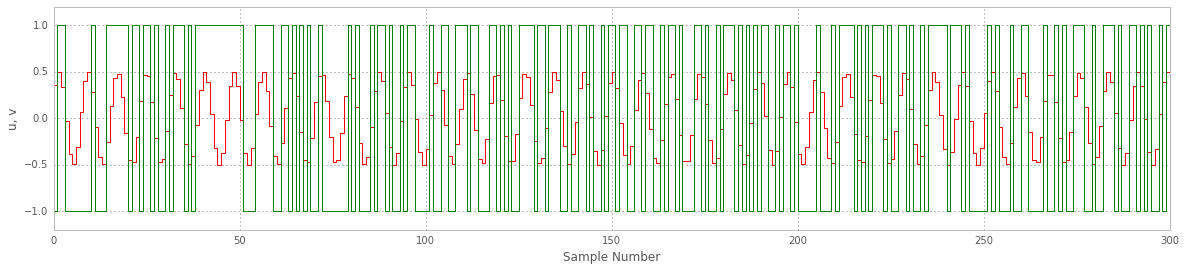### Output spectrum and extraction of the SNR with calculateSNR¶

As in the case before, from the above time simulation, the spectrum is computed - through direct FFT of the Hann-windowed time waveform.

The obtained spectrum (blue) is compared with the expected performances that can be computed evaluating the modulator transfer function in the frequency domain (magenta).

In :
spec = np.fft.fft(v*ds_hann(N))/(N/4)
plot(np.linspace(0, 0.5, N/2. + 1), dbv(spec[:N / 2 + 1]), label='Simulation')
figureMagic([0, 0.5], 0.05, None, [-140, 0], 20, None, (16, 6), 'Output Spectrum')
f1 = int(np.round((f0 - 0.25/OSR) * N))
f2 = int(np.round((f0 + 0.25/OSR) * N))
snr = calculateSNR(spec[f1:f2+1], ftest - f1)
text(0.15, -10, 'SNR = %4.1f dB @ OSR=%d)' % (snr, OSR), verticalalignment='center')
grid(True)
xlabel('Normalized Frequency')
ylabel('dBFS/NBW')
NBW = 1.5/N
Sqq = 4*evalTF(H, np.exp(2j*np.pi*f))**2/3.
hold(True)
plot(f, dbp(Sqq*NBW), 'm', linewidth=2, label='Expected PSD')
text(0.475, -90, 'NBW=%4.1E x $f_s$' % NBW, horizontalalignment='right', verticalalignment='center')
legend(loc=4);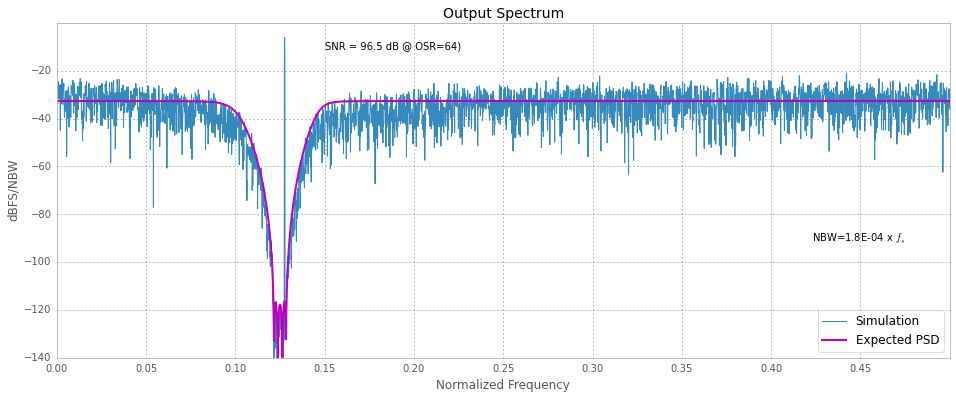### SNR vs input amplitude: prediction, simulation and peak extraction¶

In the following, the same comments as in the preceeding sections apply, adapted for band-pass frequency charactersistics.

In :
snr_pred, amp_pred, _, _, _ = predictSNR(H, OSR, None, f0)
snr, amp = simulateSNR(H, OSR, None, f0)

In :
plot(amp_pred, snr_pred, '-b', amp, snr, 'og-.')
figureMagic([-110, 0], 10, None, [0, 110], 10, None, (16, 6), 'SQNR')
xlabel('Input Level (dBFS)')
ylabel('SQNR (dB)')
pk_snr, pk_amp = peakSNR(snr, amp)
text(-20, 95, 'peak SNR = %4.1fdB\n@ OSR = %d\n' % (pk_snr, OSR), horizontalalignment='right');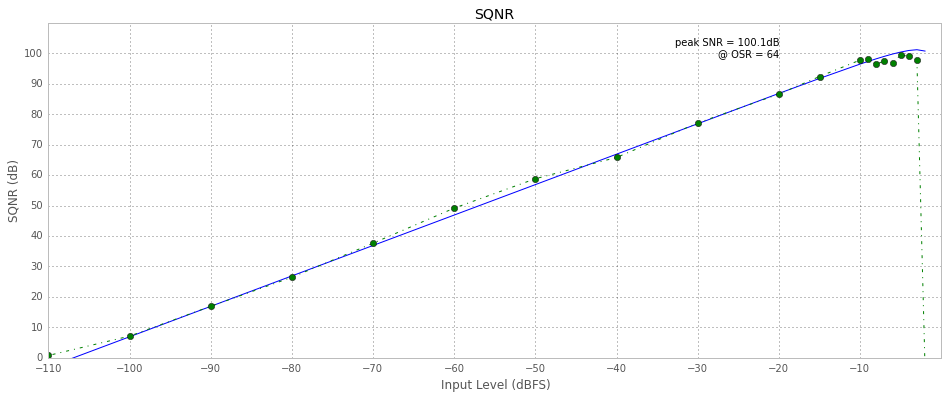## 7th-order low-pass multi-bit modulator¶

In :
figure(figsize=(16, 20))
colors = ('m', 'b')
Hinf_list = [2, 8]
i = -1
for col, Hinf in zip(colors, Hinf_list):
i += 2
OSR = 8
M = 16
H = synthesizeNTF(7, OSR, 1, Hinf)
N = 8192
fB = int(np.ceil(N/(2.*OSR)))
ftest = int(np.floor(2./7*fB))
u = 0.5*M*np.sin(2*np.pi*ftest/N*np.arange(N))
v, xn, xmax, y = simulateDSM(u, H, M + 1)

subplot(int('42' + str(i)))
t = np.arange(101)
step(t, u[t], 'g')
hold(True)
step(t, v[t], col)
figureMagic([0, 100], 10, None, [-M, M], 2, None, None,'Input & Output')
xlabel('Sample Number')
ylabel('u, v')

subplot(222)
snr, amp = simulateSNR(H, OSR, None, 0., M + 1)
plot(amp, snr,'o' + col, amp, snr, '--' + col)
hold(True)
figureMagic([-120, 0], 10, None, [0, 120], 10, None, None,'SQNR')
xlabel('Input Level (dBFS)')
ylabel('SNR (dB)')
pk_snr, pk_amp = peakSNR(snr, amp)
text(-13, pk_snr, 'peak SNR = %4.1fdB\n@ OSR = %d\n' % (pk_snr, OSR), horizontalalignment='right', color=col)

subplot(212)
f = np.linspace(0, 0.5, N/2. + 1)
spec = np.fft.fft(v*ds_hann(N))/(M*N/4)
plot(f, dbv(spec[:N/2 + 1]), col)
snr = calculateSNR(spec[2:fB + 1], ftest - 2)
text(0.1, 10*(i - 4), 'SNR = %4.1fdB @ OSR=%d' % (snr, OSR), verticalalignment='center', color=col)
figureMagic([0, 0.5], 0.05, None, [-160, 0], 20, None, None,'Output Spectrum')
xlabel('Normalized Frequency')
ylabel('dBFS')

NBW = 1.5/N
Sqq = 4*evalTF(H, np.exp(2j*np.pi*f))**2/(3.*M**2)
hold(True)
plot(f, dbp(Sqq*NBW), 'c', linewidth=2)
if i == 1:
text(0.47, -110, 'NBW=%4.1E x $f_s$ '% NBW, horizontalalignment='right')
title('15-step 7th-order Lowpass')
tight_layout()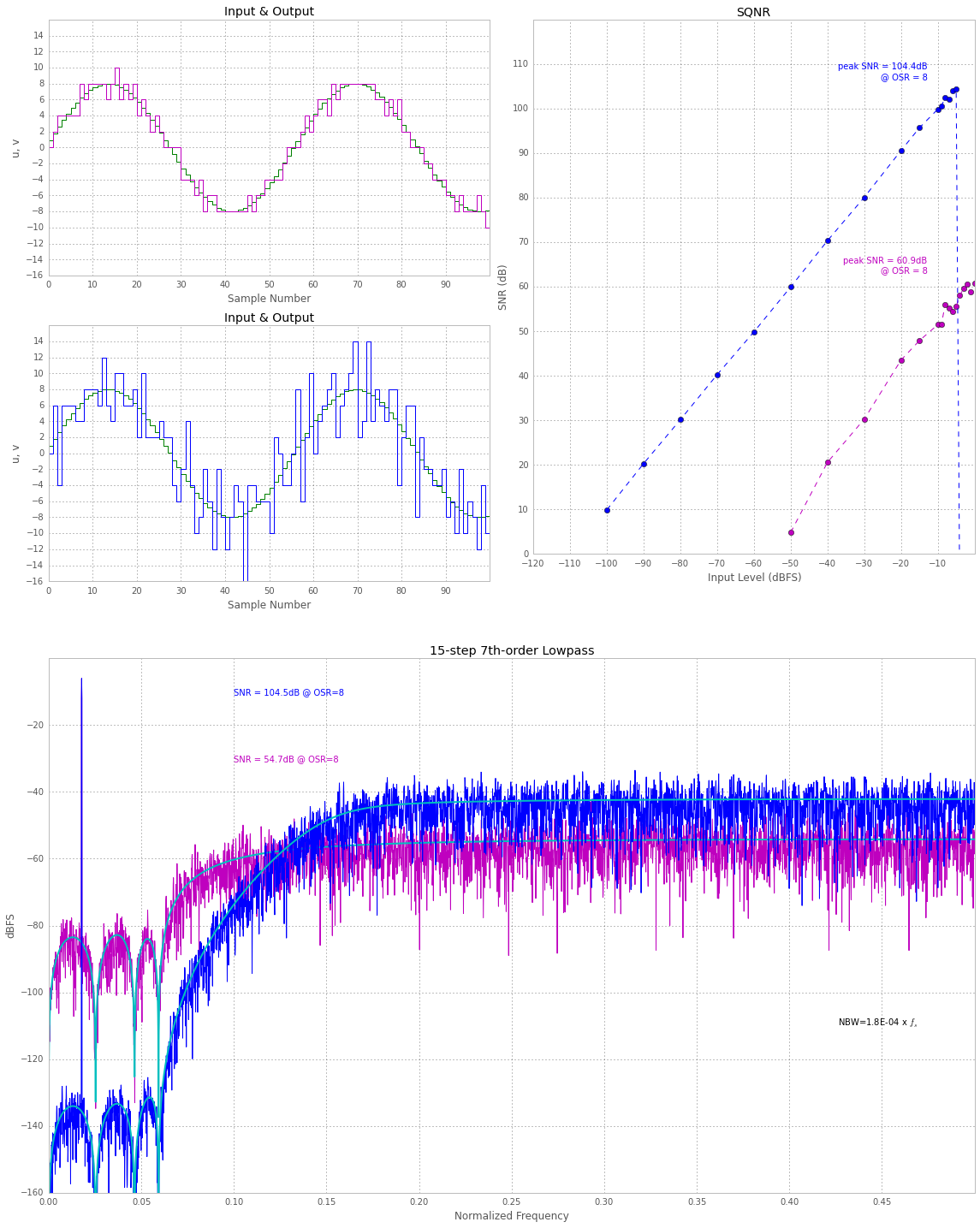## Further information about simulation of DSM's¶

Please refer to help(simulateDSM) for detailed - and possibly more updated - documentation!

### help(simulateDSM) as of writing:¶

simulateDSM(u, arg2, nlev=2, x0=0)

[v, xn, xmax, y] = simulateDSM(u, ABCD, nlev=2, x0=0)



or

[v, xn, xmax, y] = simulateDSM(u, ntf, nlev=2, x0=0)

Compute the output of a general delta-sigma modulator with input u,
a structure described by ABCD, an initial state x0 (default zero) and
a quantizer with a number of levels specified by nlev.
Multiple quantizers are implied by making nlev an array,
and multiple inputs are implied by the number of rows in u.

Alternatively, the modulator may be described by an NTF.
The NTF is a zpk object. (The STF is assumed to be 1.)
The structure that is simulated is the block-diagional structure used by
zpk2ss.

### System version information¶

In :
#%install_ext http://raw.github.com/jrjohansson/version_information/master/version_information.py